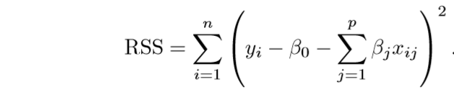It is said that regularization can help us obtain simple models over complex ones to avoid over-fitting. But for a linear classification problem:

```f(x) = Wx
```

The complexity of the model is somewhat specified: it's linear, not quadratic or something more complex. So why do we still need regularization on the parameters? Why do we prefer smaller weights in such cases?Feb 21 53 views

## 1 answer to this question.

In Machine Learning we often divide the dataset into training and test data, the algorithm while training the data can either
1) learn the data too well, even the noises which is called over fitting
2) do not learn from the data, cannot find the pattern from the data which is called under fitting.
Now, both over fitting and underfitting are problems one need to address while building models.

Regularization in Machine Learning is used to minimize the problem of overfitting, the result is that the model generalizes well on the unseen data once overfitting is minimized.
To avoid overfitting, regularization discourages learning a more sophisticated or flexible model. Regularization will try to minimize a loss function by inducing penalty.
For Example
The residual sum of squares is our optimization function or loss function in simple linear regression (RSS).Here ,

y is the dependent variable,
x1, x2, x3,..... xn are independent variables.
b0, b1 ,b2.........  bn, are the  coefficients estimates for different variables of x, these can also be called weights or magnitudes
Regularization will shrink these coefficients towards Zero,
Minimizing the loss means less error and model will be a good fit.

The way regularization can be done is by
1) RIDGE also know as L-2 Regularization
2) LASSO (Least Absolute and Selection Operator) also known as L-1 Regularizationanswered Feb 21 by
• 6,000 points

## What is the process involved in machine Learning?

Discussing this on a high level, these ...READ MORE

## What is clustering in Machine Learning?

Clustering is a type of unsupervised learning ...READ MORE

## What's the difference between the research of Machine learning and data mining?

Data mining research mainly revolves around gathering ...READ MORE

## What is the best machine learning website?

Hi@akhtar You can find lots of website which ...READ MORE

## Assumptions of Naïve Bayes and Logistic Regression

There are very few difference between Naive ...READ MORE

## Linear Discriminant Analysis vs Naive Bayes

There are no standards fixed as to ...READ MORE

## A simple explanation of Naïve Bayes Classification

Naive Bayes Classification uses probability to classify ...READ MORE